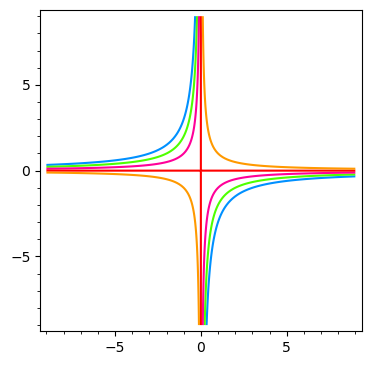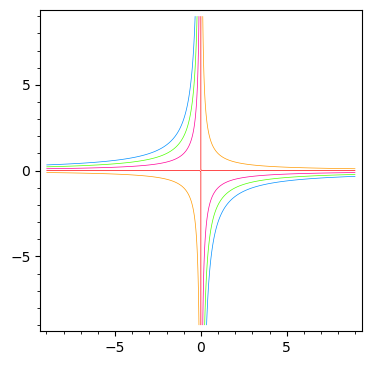# linewidth of drawhi when i type :

p(x,y)=x*y
sum(map(lambda u:implicit_plot(p-u, (-9, 9), (-9, 9),color=Color(sin(u**3/10), 1.0, 1, space='hsv')), range(-3,2)))


i would like to parameter the linewidth more fine how can i do ? the argument thickness seems not to be dealed by implicit.plot

Vincent

edit retag close merge delete

Sort by » oldest newest most voted

Use the option linewidth=0.5 in implicit_plot().

Let us illustrate the difference.

Some setup.

sage: p(x, y) = x * y
sage: xx = yy = (-9, 9)
sage: uu = range(-3, 2)
sage: opt = dict(linewidth=0.5)
sage: col = lambda u: Color(sin(u**3/10), 1.0, 1, space='hsv')


Implicit plots with default line width.

sage: sum(implicit_plot(p - u, xx, yy, color=col(u)) for u in uu)Implicit plots with line width 0.5.

sage: sum(implicit_plot(p - u, xx, yy, color=col(u), linewidth=0.5) for u in uu)more